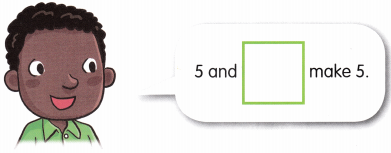Count and write.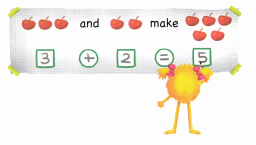Question 1.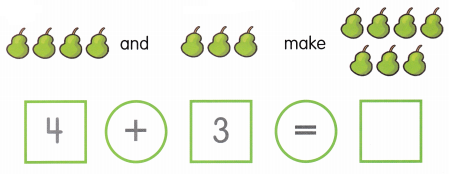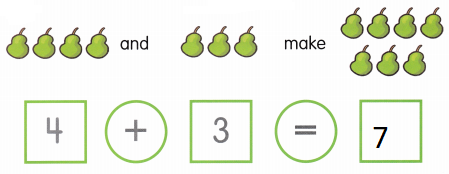Explanation:
The sum of  pears are 7
4 + 3 = 7

Question 2.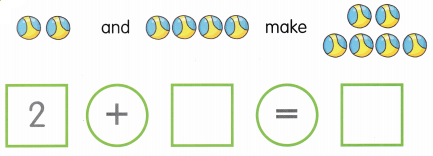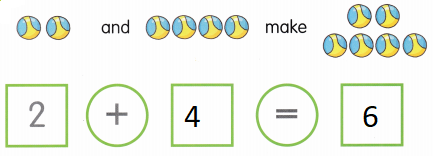Explanation:
The sum of  balls are 6
2 + 4 = 6

Count and write.

Question 3.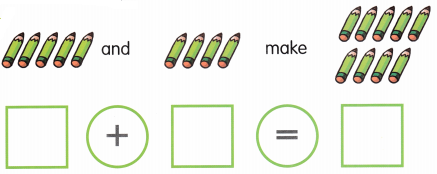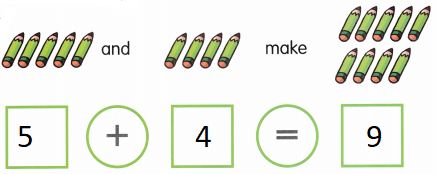Explanation:
The sum of  pencils are 9
5 + 4 = 9

Question 4.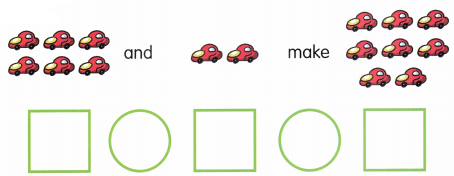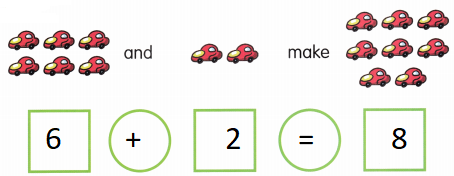Explanation:
The sum of  cars are 8
6 + 2 = 8

Question 5.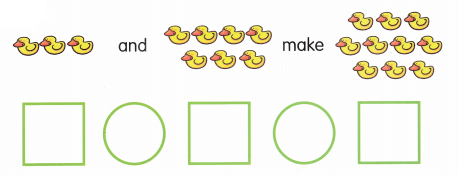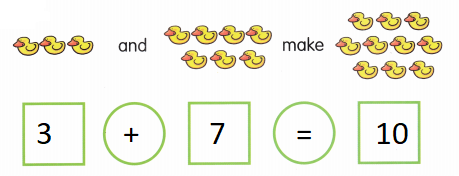Explanation:
The sum of  ducks are 10
3 + 7 = 10

Count and write.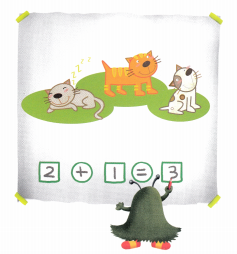Question 1.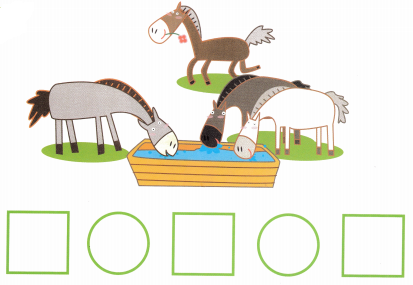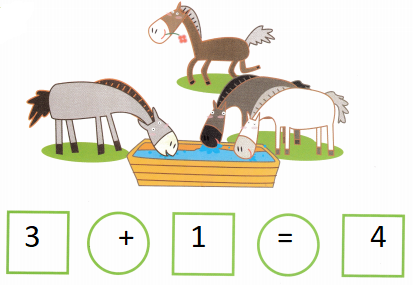Explanation:
The sum of animals drinking the water and not drinking the water
3 + 1 = 4

Question 2.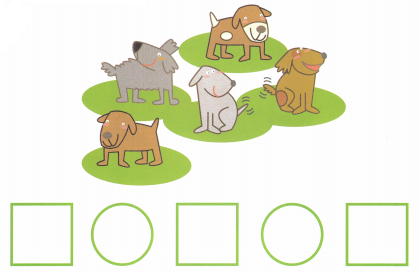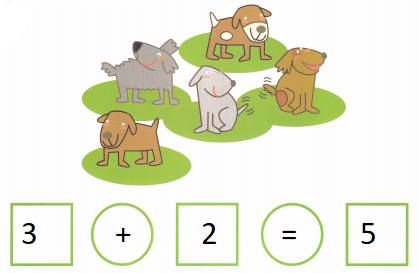Explanation:
The sum of brown dogs and grey dogs in the park are 5
3 + 2 = 5

Question 3.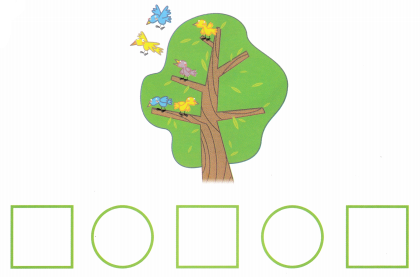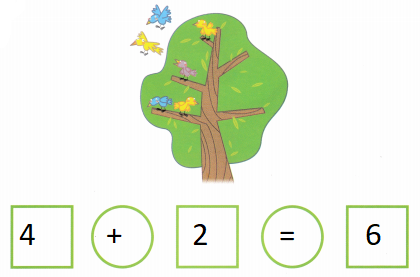Explanation:
The sum of  birds on the tree and the birds on the sky are 6
4 + 2 = 6

Question 4.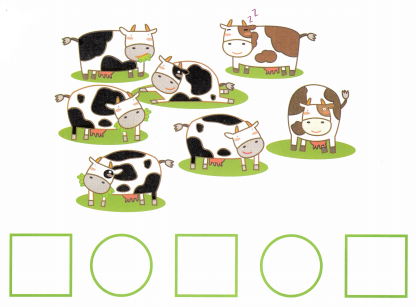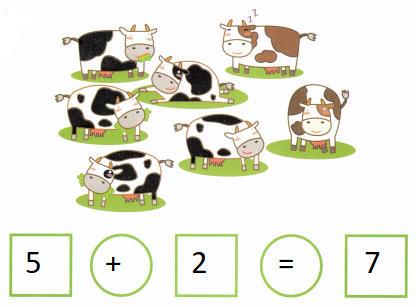Explanation:
The sum of  brown cows and black cows are 7
5 + 2 = 7

Question 5.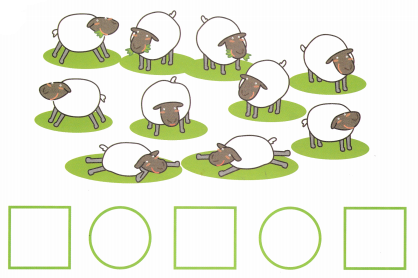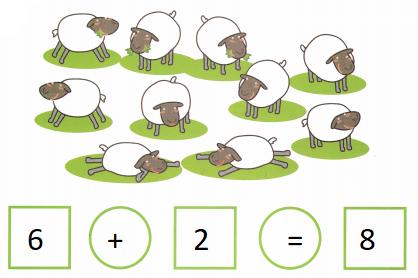Explanation:
The sum of  sleeping sheep’s and standing sheep’s are 8
6 + 2 = 8

Lesson 2 Addition Facts to 5

Fill in the missing numbers.

Question 1.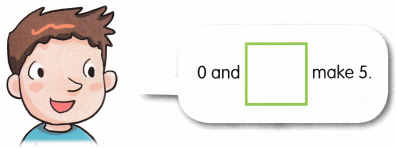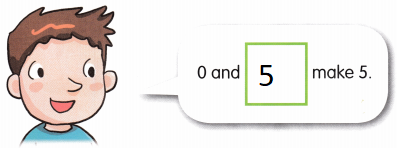Explanation:
When we add 0 to a number than the result is the same number.
Hence 0 is called the additive identity.
The sum of 0 and 5 is 5

Question 2.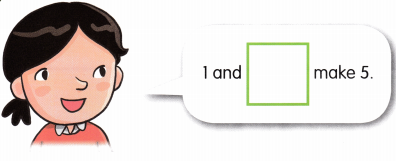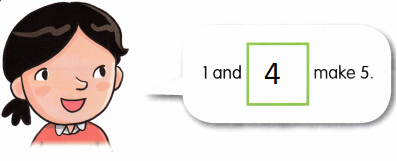Explanation:
The sum of 1 and 4 makes 5
1 + 4 = 5

Question 3.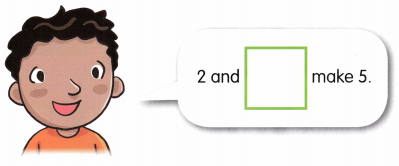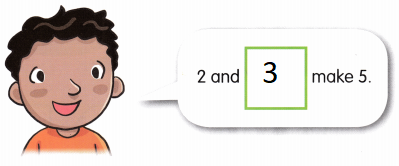Explanation:
The sum of 2 and 3 makes 5
2 + 3 = 5

Question 4.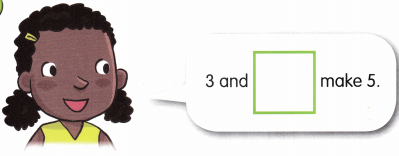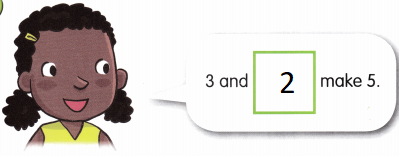Explanation:
The sum of 3 and 2 makes 5
3 + 2 = 5

Question 5.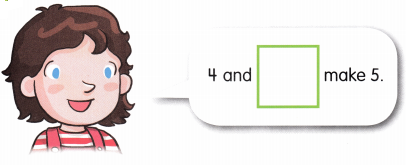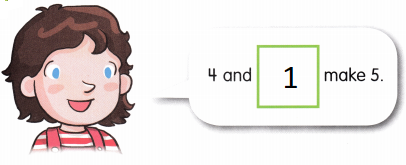Explanation:
When we add 0 to a number than the result is the same number.
Hence 0 is called the additive identity.